Download Presentation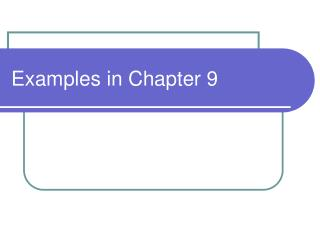Examples in Chapter 9

# Examples in Chapter 9 - PowerPoint PPT Presentation

Examples in Chapter 9 . 9.25. A flywheel with a radius of 0.3 m starts from rest and accelerates with a constant angular acceleration of 0.6 rad/s 2 . Compute the magnitude of the angular accleration the radial acceleration The resultant acceleration of a point on its rim At the startI am the owner, or an agent authorized to act on behalf of the owner, of the copyrighted work described.
Download Presentation## Examples in Chapter 9

An Image/Link below is provided (as is) to download presentation

Download Policy: Content on the Website is provided to you AS IS for your information and personal use and may not be sold / licensed / shared on other websites without getting consent from its author.While downloading, if for some reason you are not able to download a presentation, the publisher may have deleted the file from their server.

- - - - - - - - - - - - - - - - - - - - - - - - - - E N D - - - - - - - - - - - - - - - - - - - - - - - - - -
Presentation Transcript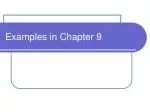### Examples in Chapter 9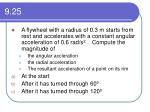9.25
• A flywheel with a radius of 0.3 m starts from rest and accelerates with a constant angular acceleration of 0.6 rad/s2 . Compute the magnitude of
• the angular accleration
• the radial acceleration
• The resultant acceleration of a point on its rim
• At the start
• After it has turned through 600
• After it has turned through 1200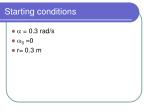Starting conditions
• a = 0.3 rad/s
• w0 =0
• r= 0.3 m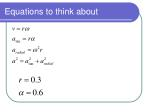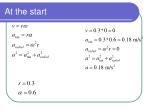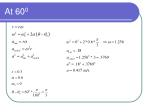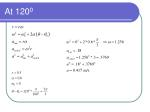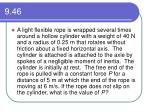9.46
• A light flexible rope is wrapped several times around a hollow cylinder with a weight of 40 N and a radius of 0.25 m that rotates without friction about a fixed horizontal axis. The cylinder is attached is attached to the axle by spokes of a negligible moment of inertia. The cylinder is initially at rest. The free end of the rope is pulled with a constant force P for a distance of 5 m at which the end of the rope is moving at 6 m/s. If the rope does not slip on the cylinder, what is the value of P?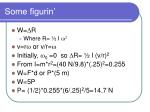Some figurin’
• W=DR
• Where R= ½ I w2
• v=rw or v/r=w
• Initially, w0 =0 so DR= ½ I (v/r)2
• From I=m*r2=(40 N/9.8)*(.25)2=0.255
• W=F*d or P*(5 m)
• W=5P
• P= (1/2)*0.255*(6/.25)2/5=14.7 N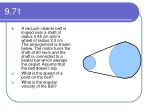9.71
• A vacuum cleaner belt is looped over a shaft of radius 0.45 cm and a wheel of radius 2.0 cm . The arrangement is shown below. The motor turns the shaft at 60 rev/s and the shaft is connected to a beater bar which sweeps the carpet. Assume that the belt doesn’t slip.
• What is the speed of a point on the belt?
• What is the angular velocity of the belt?Some more figurin’
• Part a) v=r*w where
• r=0.45 cm
• w= 60 rev/s *(2*p radians/rev)=377 rad/s
• v=0.45*377=169 cm/s or 1.69 m/s
• Part b) w2= 169 cm/s / 2 cm =84.8 rad/sHint on 9.72
• The wheels are coupled so that there is the tangential velocity is constant so that

w1/w2 = r2/r1